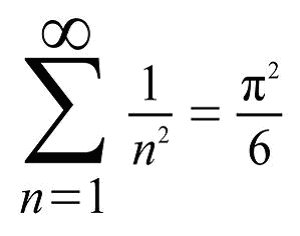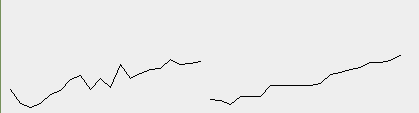# Computation Contest #7 Results and Solution## Solution

The problem of this contest was to test two methods to approximate pi and measure how accurate they get compared to how many digits it gets right.
I wrote a simple code which uses the following two methods:

1. Count how many grid dots are in a circle(can be done in linear time when using the circle function).
2. Use the following sequence to apprixmate it:I decided to go with a maximum accuracy of twenty binary digits. Here is my code

``````import javax.swing.JFrame;
import java.awt.Graphics;
import java.awt.Color;

public class abcd extends JFrame {
int[] firstMethod;
int[] secondMethod;
public abcd() {
setSize(800, 400);
setVisible(true);

doFirstMethod();
doSecondMethod();
repaint();
}
// return accuracy in bits:
public int getAccuracy(double approx) {
if((int)approx != 3)
return 0;
long approxL = Double.doubleToLongBits(approx);
long piL = Double.doubleToLongBits(Math.PI);
long mantissaStart = 0x0008000000000000L;
int prec = 0;
for(long i = mantissaStart; i >= 0; i >>= 1) {
if((approxL & i) != (piL & i))
return prec;
prec++;
}
return prec;
}
public void doFirstMethod() {
firstMethod = new int;
int index = 0;
for(int i = 0; index < 20; i += 1+(i>>3)) {
double π = countDots(i);
int numDigits = getAccuracy(π);
if(numDigits >= index+1) {
// Repeat the calculation a couple of times to increase time accuracy
long t1 = System.nanoTime();
for(int j = 0; j < 1000000; j++)
getAccuracy(π);
long t2 = System.nanoTime();
firstMethod[index] = (int)((t2-t1)/1000000.0);
index++;
}
}
}
public void doSecondMethod() {
secondMethod = new int;
int index = 0;
for(int i = 0; index < 20; i += 1+(i>>3)) {
double π = sumUp(i);
int numDigits = getAccuracy(π);
if(numDigits >= index+1) {
// Repeat the calculation a couple of times to increase time accuracy
long t1 = System.nanoTime();
for(int j = 0; j < 1000000; j++)
getAccuracy(π);
long t2 = System.nanoTime();
secondMethod[index] = (int)((t2-t1)/1000000.0);
index++;
}
}
}

// Use the partial sums of the infinite sequence sum 1/n² = π²/6:
public double sumUp(int n) {
double sum = 0;
for(int i = 1; i <= n; i++) {
sum += 1.0/i/i;
}
return Math.sqrt(sum*6);
}

// Counts how many grid points lie in-/outside a quarter circle. Kind of similar to integrating the circle function.
public double countDots(int r) {
long num = 0;
long rSqr = r*r;
for(int i = 0; i < r; i++) {
num += (long)(Math.sqrt(rSqr-i*i)); // Use the floor function to find the number of grid points inside the circle at this position.
}
return 4*num/(double)rSqr;
}

public void paint(Graphics g) {
g.setColor(Color.BLACK);
for(int i = 1; i < 20; i++) {
g.drawLine(i*10, 200-firstMethod[i-1], i*10+10, 200-firstMethod[i]);
g.drawLine(200+i*10, 200-secondMethod[i-1], 200+i*10+10, 200-secondMethod[i]);
}
}

public static void main(String[] args) {
new abcd();
}
}
``````

This code outputs the following two graphs:Not much to see except from the fact that both graphs are growing kind of linear.
↓↑↓↑↓↑↓↑↓↑↓↑↓↑↓↑↓↑↓↑↓↑↓↑↓↑↓↑↓↑↓↑↓↑↓↑↓↑↓↑↓↑↓↑↓↑↓↑↓

### List of participants with their entries:

NameSolution foundComment
@lalloIntegrating the circle functionStrange that your function seems to grow exponentially, while mine are at least on the scale I observed mostly linear. Probably python is doing something strange?

↓↑↓↑↓↑↓↑↓↑↓↑↓↑↓↑↓↑↓↑↓↑↓↑↓↑↓↑↓↑↓↑↓↑↓↑↓↑↓↑↓↑↓↑↓↑↓↑↓

## Winners:

Congratulations @lallo, you won 2 SBI!

↓↑↓↑↓↑↓↑↓↑↓↑↓↑↓↑↓↑↓↑↓↑↓↑↓↑↓↑↓↑↓↑↓↑↓↑↓↑↓↑↓↑↓↑↓↑↓↑↓

### The next contest starts tomorrow. Don't miss it!

0
0
0.000A member bonus \$trendotoken tip, and !trendovoter, for @quantumdeveloper from MAPXV! These bonuses are for members, selected at random, and last for a few days.

Also consider our MAPR fund and MAXUV vote bonds too.
MAP Steem Fintech: growing your STEEM without SP.

0
0
0.000A bonus \$trendotoken tip from ONECENT!
Also consider MAPR fund and MAXUV vote bonds too.
MAP Steem FinTech: growing your STEEM without SP.

0
0
0.000I think in my case it grows exponentially because my algorithm doesn't stop when a certain level of precision is achieved, but it stops after doing n iterations.
So it depends on the input that is exponential. But I'm not sure.
I should modify the algorithm and find the exactly number of iteration and time needed to achieve 1,2,3,4,5,... decimal digits correct. And see if it changes.

0
0
0.000